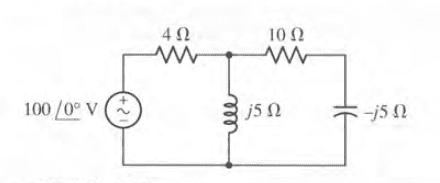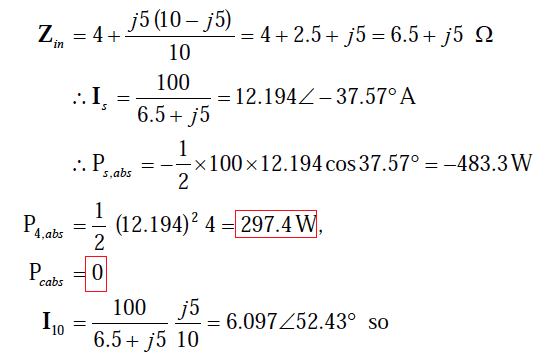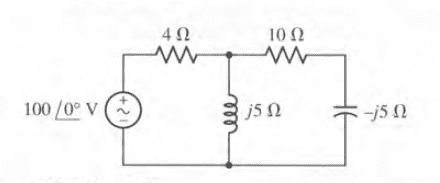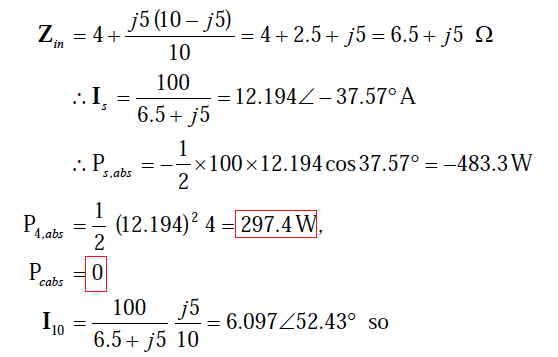# Voltage Division - AC cct.

## Homework Statement

Hi,
We have this cut. :and in the image below, I10 is calculated by using current division :## The Attempt at a Solution

I tried to find it using voltage division but the answer is wrong :
Vx(of the upper node) = 100 * $$\frac{j5}{4+j5}$$ = 60.98 + j48.78 V

=> I10 = $$\frac{Vx}{10 - j5}$$ = 2.93 + 6.34 = 6.98 $\ 65.2$

which is wrong, WHY?

thanks

gneill
Mentor

## Homework Statement

Hi,
We have this cut. :and in the image below, I10 is calculated by using current division :## The Attempt at a Solution

I tried to find it using voltage division but the answer is wrong :
Vx(of the upper node) = 100 * $$\frac{j5}{4+j5}$$ = 60.98 + j48.78 V

=> I10 = $$\frac{Vx}{10 - j5}$$ = 2.93 + 6.34 = 6.98 $\ 65.2$

which is wrong, WHY?
Your voltage division isn't taking into account the impedance of the 10Ω and -5j capacitor branch that's also connected at the Vx node. You might try applying nodal analysis to find Vx...

Last edited:
oh
thank you gneill